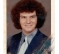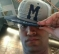# Polynomial calculations on an FIR filter engine, part 1

Polynomial evaluation is structurally akin to FIR filtering and fits dedicated filtering engines quite well, with certain caveats. It’s a technique that has wide applicability. This two-part note discusses transducer and amplifier non-linearity compensation, function approximation and aspects of harmonic signal synthesis.

Need for polynomials as general non-linear functions

Many transducer types exhibit a non-linear relationship between a measured parameter, such as a voltage, and the underlying physical quantity whose value is desired. The measurement system has to calculate the value of a non-linear function. This can sometimes put significant additional burden on the CPU in the measurement system – though modern CPUs generally have sufficient horsepower that this is not an issue at the slow reading rates often encountered.

In higher-grade data acquisition systems the reading rate may start to stress the available processor resources. Fast, accurate calculation of the desired quantities in hardware can be a valuable addition to such systems. FIR filtering engines, such as the Digital Filter Block in Cypress Semiconductor’s PSoC3 and PSoC 5LP families, can calculate typical non-linear correction formulae rapidly and accurately, provided that certain guidelines are followed.

The nonlinearity correction is nearly always achieved through the evaluation of a polynomial in the measured value (or a scaled and shifted version of it). Polynomials are simple yet flexible ways of representing functions, and can be evaluated on primitive hardware lacking built-in routines for major functions. There are several cases to consider:

1- The correction function is available in closed form; it isn’t a polynomial, but it is expedient to use a well-behaved polynomial approximation to simplify the calculation.

The most common example is the use of the binomial approximation to division.  Simple FIR filter engines architectures such as the Cypress DFB don’t have a division instruction or significant bit-manipulation capability, and full-precision long division is computationally very expensive.

Binomial division relies on the expansion of the dividend:

$$\frac{1}{1+x} = 1 - x + x^2 - x^3 ... \tag{1}$$

producing a polynomial that can be evaluated using only multiplies and adds.

One example use of this is in evaluating formulae that are themselves approximations.  For instance, to quickly calculate an approximation to 2x on an engine such as the DFB, the input variable x is first shifted to a value y in the range (0,1).  The number of bits N shifted gives the first factor in the answer, 2N.  The fractional component 2y is approximated as

$$2^y \approx \frac{3+y}{3-y} \tag{2}$$

which is accurate to about 1% in the range $[0 \lt y \lt 1]$. This accuracy is more than good enough for, for instance, a high dynamic range light exposure meter using a logarithmically responding input TIA.

Equation  can be scaled and approximated with  to give

$$2^y \approx \frac{3+y}{3-y} = \frac{1+\frac{y}{3}}{1-\frac{y}{3}}$$

$$i.e. 2^y \approx (1+\frac{y}{3})(1+\frac{y}{3}+\frac{y^2}{9}+\frac{y^3}{27}+\frac{y^4}{81}+...) \tag{3}$$

$$2^y \approx 1+\frac{2y}{3}+\frac{2y^2}{9}+\frac{2y^3}{27}+\frac{2y^4}{81}+\frac{y^5}{243} \tag{4}$$

and equation  gives enough terms to not significantly degrade accuracy. In fact, this is wasteful of terms. If an approximating polynomial is directly optimized in a spreadsheet, better results can be achieved. For example:

$$2^y \approx 1+\frac{5y}{8}+(\frac{5y}{8})^2 \tag{5}$$

is accurate to ~0.7% in the range $[0 \lt y \lt 1]$, and has a form that’s particularly easy to compute, as we’ll see in a later section. Allowing free selection of the two coefficients can get the error down to around 0.2%.

Another example using approximated division is bridge linearization.  Some bridge configurations have a non-linear relationship between bridge output voltage and the bridge element’s fractional parameter change.  The expression to calculate has a very simple form but it is again rational and requires a division.  The number of terms from the binomial expansion to be evaluated is decided from the maximum value of bridge output voltage, relative to excitation, that needs to be supported at a given accuracy.

The dividend is scaled so that the binomial series is infinitely convergent.  In this form of polynomial, the value of each successive power term is less than the one before, which makes data scaling simple and eases the use of a fixed-point architecture.  That’s not necessarily the case in the next class of problem.

2- The correction function isn’t available in closed form, but extensive measurements have been subjected to a polynomial fit by a third party, which allows accurate interpolation with a bounded error.

An example of this is thermocouple linearization.  Standard expressions are provided by NIST that evaluate up to a ninth-order polynomial in the measured thermocouple voltage.  The coefficients for these polynomials are generated by curve-fitting programs.  The values of each of the computed power terms can vary widely, and the final value is achieved through the cancellation of multiple terms whose individual values are much larger.  Significant arithmetic precision is often required in the evaluation of such polynomials, and it’s usually assumed that floating point arithmetic will be used.  Fixed-point arithmetic can be used in such cases, after a careful study to find the data scaling arrangement that minimizes loss of precision.

This issue is common whenever Chebychev approximation techniques have been used on tabulated data.  The Chebychev approximation is close to the minimum possible approximation error for a given polynomial order, but this relies on very high precision cancellation of calculated values.  This is evident in the thermocouple linearization work where extreme care is needed in coding, even with double precision floating point arithmetic.

Fixed-point engines such as the DFB will not be able to produce the same number as high precision floating-point arithmetic.  But in the majority of cases it will be able to produce results whose difference is completely inconsequential.

3- The correction function is determined indirectly through harmonic analysis of a sinewave input.

One application for this is in linearity correction of an imperfect amplifier.  Harmonic distortion measurements can be used to determine the transfer function linearity of the amplifier.  This could be an audio amplifier, a communication line driver or an RF power amplifier.  This works best when the amplifier is operated in a region where its performance is not strongly dependent on input frequency, or for which the frequency dependence can be robustly modeled.  Otherwise, more complex techniques such as Volterra series analysis are needed, and that’s beyond the scope of this note.

The system is probed with sinusoidal test inputs and the value of higher order harmonics is measured as a function of amplitude.  These harmonic terms are usually small in value compared to the main signal.  Because raising a sinewave to the nth power creates all harmonics up to the nth, a set of equations is solved to determine the required polynomial coefficients.  While the value of the power terms doesn’t necessarily decrease monotonically, practical experience shows that there’s negligible danger that intermediate results will ‘blow up’.

A second, similar application of this is in direct additive synthesis of waveforms, for instance in sound synthesis.  If the spectral makeup of a waveform is known, it can be recreated by passing a sinewave signal through a specific non-linearity, represented as a polynomial.  The method has a few limitations; it is very hard to generate sin(nx) from polynomial expansions of either purely sin(x) or purely cos(x).  So, if the phase of the harmonics is also important, this is not the most flexible way to go.  There’s a Filter Wizard article covering this.

Polynomial evaluation on FIR filter engines

The native signal input range of fixed-point engines such as the Cypress DFB is typically from -1 to slightly less than +1, so all input data needs to be scaled into this range.  This is the natural format from the output of a bipolar ratiometric ADC.  Coefficients also need to be scaled to fit into this range.  This means that the filter engine will typically only calculate the required result to within a known constant factor.  By arranging for this factor to equal a power of two, the final scaling stage is made simple.

Horner’s method is commonly used to evaluate polynomials.  It relies on the rewriting of

$$H(x) = a_0+a_1x+a_2x^2+a_3x^3+...+a_nx^n \tag{6}$$

as

$$H(x) = a_0+x(a_1+x(a_2+x(a_3+...x(0+a_n)...))) \tag{7}$$

Evaluation of  begins at the deepest level of parenthesis. In pseudocode the evaluation proceeds something like this:

clear accumulator
loop
MAC A,B
ACC B
write accumulator into B
write accumulator to output


When the loop falls through, all the coefficients have been multiplied in. Each computation requires N+1 MACs for an nth order polynomial.For a 9th order polynomial, an engine like the DFB can finish this in about twenty system clock cycles, plus some change for data management. In contrast, the 8051 CPU requires some 8000 clock cycles to execute this calculation in floating point C.

One disadvantage of Horner’s method on a filter engine is that the value of the accumulator at any stage of the calculator must not be allowed to overflow. If we were just multiplying up independent terms and adding them, accumulator overflow could be tolerated as long as the final answer of a perfect calculation will fit into the accumulator. With a nested calculation like this, though, we cannot recover from the drastic loss of integrity that occurs when the accumulator contents don’t fit.

To make Horner’s method safe from overflow, all the coefficient values must be checked in advance, using an algorithm that recursively calculates the maximum possible value that the accumulator can reach. This only needs to be done at coefficient design time, so it does not affect the time performance of the algorithm. However, the necessary coefficient scaling will significantly reduce the precision available, especially in case (2) above where there is a lot of cancellation going on between different terms.

It’s usually considered a cardinal sin to compute a polynomial the brute-force way, as written in . This is because more multiplications are needed, and also because, in the general case where x>>1, the limits of machine precision are easily breached. However, for modest polynomial evaluations on a filter engine, it can be a valid alternative. There are often plentiful machine cycles available to do the extra ‘wasteful’ multiplies. Also, all data and coefficients are <1, meaning that the terms are guaranteed to individually fit within the fractional number space of such an engine. Precision is lost through the iterative calculation of powers of x. But no further loss occurs during the summation of the terms of  since they are all MAC’d into the accumulator like a little FIR filter, with no truncation and no worry about overflow as long as we know (by design) that the final answer must fit.

The choice of whether to use the direct or Horner methods can be determined by an analysis of the coefficients in the particular case. In part 2 of this note we’ll look at a typical polynomial application, the linearization (i.e. conversion to temperature) of a thermocouple’s output signal.

[ - ]
Comment by October 18, 2019Hi Kendall,

In the paragraph just after equation (1) you discuss computing 2^x. First you determine y = x >> N, where N = floor(x), or the integer portion of x. Then you discuss how to compute 2^y.

Why do we want to compute 2^y? I would think we want to compute 2^(x - N), i.e., the fractional part of x.

--Randy

[ - ]
Comment by March 2, 2021Thank you very much for the post.

How can I find part 2? It was better if you put it at the end of this post.

Bests,

To post reply to a comment, click on the 'reply' button attached to each comment. To post a new comment (not a reply to a comment) check out the 'Write a Comment' tab at the top of the comments.